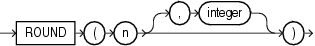# ROUND (number)

Syntax

round_number::=Description of the illustration round_number.gif

Purpose

`ROUND` returns `n` rounded to `integer` places to the right of the decimal point. If you omit `integer`, then `n` is rounded to 0 places. The argument `integer` can be negative to round off digits left of the decimal point.

`n` can be any numeric datatype or any nonnumeric datatype that can be implicitly converted to a numeric datatype. The argument `integer` must be an integer. If you omit `integer`, then the function returns the same datatype as the numeric datatype of the argument. If you include `integer`, then the function returns `NUMBER`.

For `NUMBER` values, the value `n` is rounded away from 0 (for example, to `x`+1 when `x`.5 is positive and to `x`-1 when `x`.5 is negative). For `BINARY_FLOAT` and `BINARY_DOUBLE` values, the function rounds to the nearest even value. Refer to the examples that follow.

Table 2-10, "Implicit Type Conversion Matrix" for more information on implicit conversion

Examples

The following example rounds a number to one decimal point:

```SELECT ROUND(15.193,1) "Round" FROM DUAL;

Round
----------
15.2
```

The following example rounds a number one digit to the left of the decimal point:

```SELECT ROUND(15.193,-1) "Round" FROM DUAL;

Round
----------
20
```

The following examples illustrate the difference between rounding `NUMBER` and floating-point number values. `NUMBER` values are rounded up (for positive values), whereas floating-point numbers are rounded toward the nearest even value:

```SELECT ROUND(1.5), ROUND(2.5) FROM DUAL;

ROUND(1.5) ROUND(2.5)
---------- ----------
2          3

SELECT ROUND(1.5f), ROUND(2.5f) FROM DUAL;

ROUND(1.5F) ROUND(2.5F)
----------- -----------
2.0E+000    2.0E+000
```